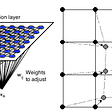# [ML Crash Course] Training and Reducing Loss: An Iterative Approach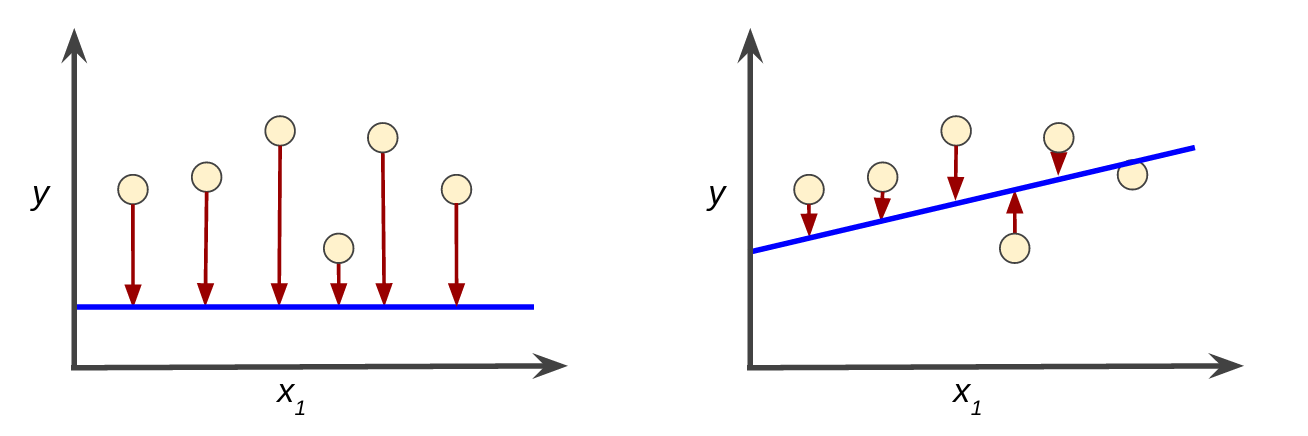High loss in the left model; low loss in the right model

Training a model simply means determining (learning) good values for all the weights and the bias from labeled examples. In supervised learning, a machine learning algorithm builds a model by examining many examples and attempting to find a model that minimizes loss.

Loss is the penalty for a bad predition. That is, the loss is a number indicating how bad the model’s prediction was on a single example. If the model’s prediction is perfect, the loss is zero; otherwise, the loss is greater. The goal of training a model is to find a set of weights and biases that have low loss, on average, across all examples. For example, the following figure shows a high loss model on the left and a low loss model on the right. Note the following about the figure:

• The circles are examples (features and given label).
• The blue lines represent predictions from models
• The arrows represent a loss.High loss in the left model; low loss in the right model

Notice that the arrows in the left plot are much longer than their counterparts in the right plot. Clearly, the line in the right plot is a much better predictive model than the line in the left plot.

You might be wondering whether you could create a mathematical function — a loss function — that would aggregate the individual losses in a meaningful fashion.

# Squared loss: a popular loss function

The linear regression models we’ll examine here use a loss function called squared loss (also known as L2 loss).

Mean square error (MSE) is the average squared loss per example over the whole dataset. To calculate MSE, sum up all the squared losses for individual examples and then divide by the number of examples:

where:

• (x, y) is an example in which x is the set of features (for example, Father’s height, age, gender) that the model uses to make predictions and y is the example’s label (for example, Son’s height).
• prediction(x) is a function of the weights and bias in combination with the set of features x.
• D is a data set containing many labeled examples, which are (x, y) pairs.
• N is the number of examples in D.

Although MSE is commonly-used in machine learning, it is neither the only practical loss function nor the best loss function for all circumstances.

# Reducing Loss: An Iterative Approach

In the iterative learning, you’ll start with a wild guess (“The value of w₁ is 0.”) and wait for the system to tell you what the loss is. Then, you’ll try another guess (“The value of w₁ is 0.5.”) and see what the loss is. The real trick to the game is trying to find the best possible model (w₁ and b, for example) as efficiently as possible.

The following figure suggests the iterative trial-and-error process that machine learning algorithms use to train a model:

The “model” takes one or more features as input and returns one prediction (y′) as output. To simplify, consider a model that takes one feature and returns one prediction:

What initial values should we set for b and w₁? For linear regression problems, it turns out that the starting values aren’t important. We could pick random values, but we’ll just take the following trivial values instead:

• b = 0
• w₁ = 0

Suppose that the first feature value is 10. Plugging that feature value into the prediction function yields:

The “Compute Loss” part of the diagram is the loss function that the model will use. Suppose we use the squared loss function. The loss function takes in two input values:

• y′: The model’s prediction for features x
• y: The correct label corresponding to features x.

At last, we’ve reached the “Compute parameter updates” part of the diagram. It is here that the machine learning system examines the value of the loss function and generates new values for b and w₁. For now, just assume that this mysterious box devises new values and then the machine learning system re-evaluates all those features against all those labels, yielding a new value for the loss function, which yields new parameter values. And the learning continues iterating until the algorithm discovers the model parameters with the lowest possible loss. Usually, you iterate until overall loss stops changing or at least changes extremely slowly. When that happens, we say that the model has converged.

Iterative strategies are prevalent in machine learning, primarily because they scale so well to large data sets. A Machine Learning model is trained by starting with an initial guess for the weights and bias and iteratively adjusting those guesses until learning the weights and bias with the lowest possible loss.

--

--

--

## More from A Ydobon

Ydobon is nobody.

Love podcasts or audiobooks? Learn on the go with our new app.

## No PhD? No problem! Machine Learning for the rest of us## The Secret to Mastering ML## Writing your first Machine Learning Model from Scratch.## An Introduction to Neural Networks with Implementation from Scratch using Python## Introduction to Visual SLAM: Chapter 1 —Introduction to SLAM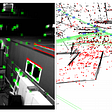## Image Classification with Ensemble of Deep Neural Networks using TensorFlow + TPUs## Responsible and Ethical Design of Machine Learning-Based Products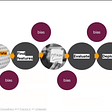## A Ydobon

Ydobon is nobody.

## Regularization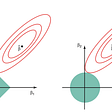## Procrastination: Things we plan to do later are mostly those we will never do….## Kohonen Neural Networks : Intuition